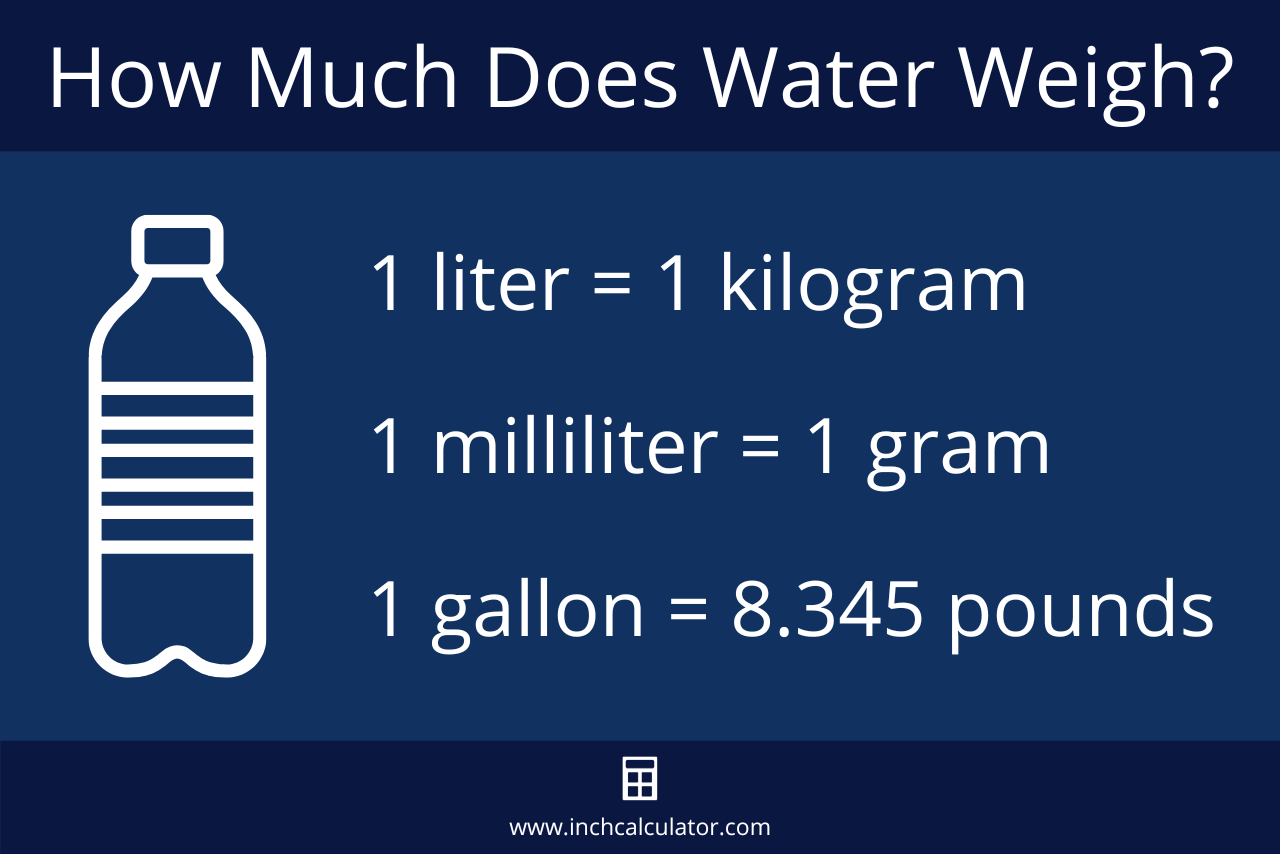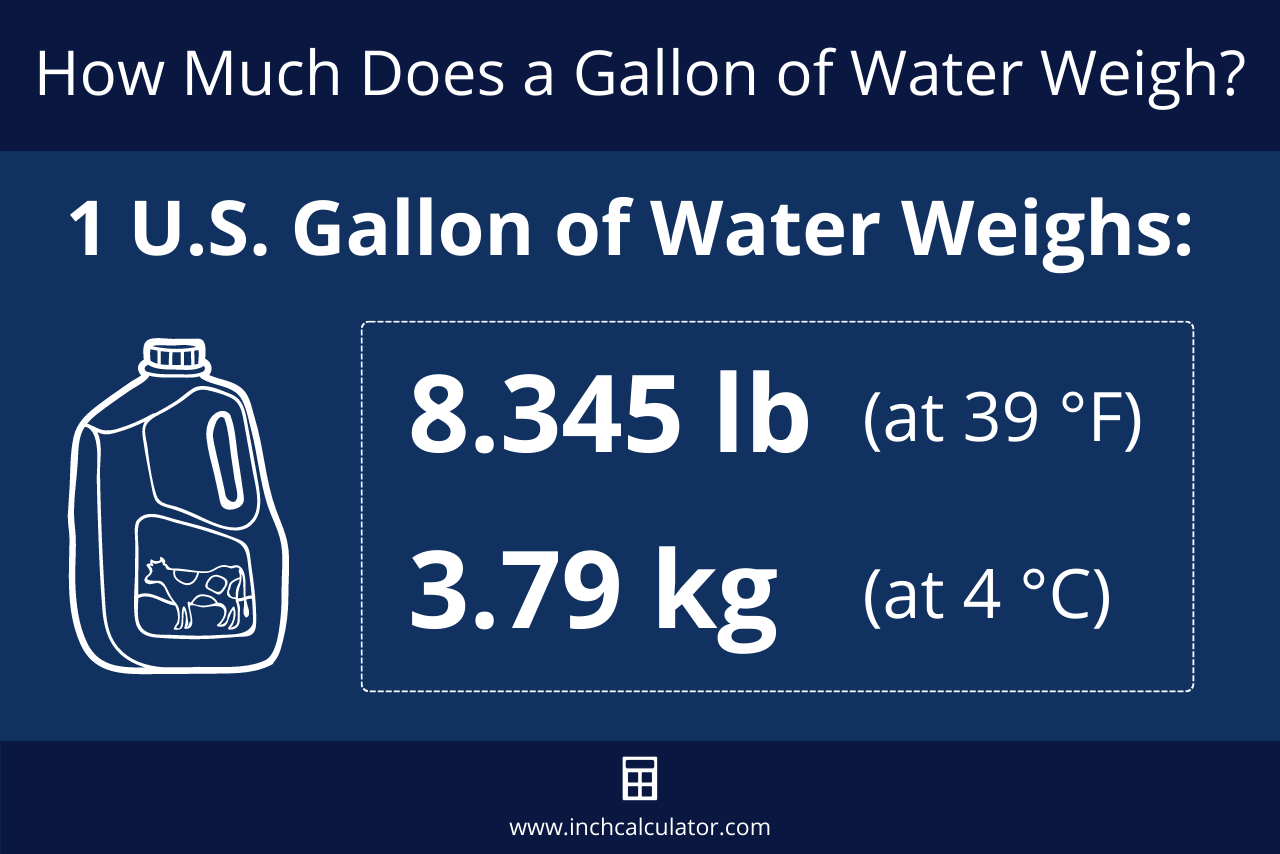# Water Weight Calculator

Find how much water weighs given a volume in teaspoons, tablespoons, cups, quarts, pints, gallons, liters, or milliliters.

## Results:

133.53
Ounces
8.345
Pounds
3,785.4
Grams
3.785
Kilograms
Weight at 39.2 °F (4 °C)
Learn how we calculated this below

## How Much Does Water Weigh?

The weight of a volume of water can be found given the density, which is the mass per unit volume. The density of water is 1 kilogram per liter (kg/L) at 39.2° F or 4° C, but the precise density depends on the temperature.

1 kg/L is typically used to represent the density or weight of water at normal temperatures above freezing when very precise measurements are not required.Therefore, 1 liter (L) of water weighs 1 kilogram (kg) and 1 milliliter (mL) of water weighs 1 gram (g).

In common US measures, one gallon of water weighs 8.345 pounds.

The density of water varies slightly at different temperatures, which affects the precise weight per volume.

### How to Calculate the Weight of Water

To find the weight of water, start by using the standard density (1 kg/L at 39.2°) and the volume of water in liters. Multiply the volume of water in liters by the density to find the weight.

You can use our volume converter to convert different volumes to liters.

For example: let’s find the weight of 250 mL of water.

Step one: convert volume to liters:

Volume (L) = 250 mL ÷ 1000 = 0.25 L

Step two: using the density of 1 kg/L, calculate the weight of 0.25 L of water:

Weight (g) = 0.25 L × 1 kg/L = 0.25 kg = 250 g

Thus, 250 mL of water weighs 0.25 kilograms or 250 grams.

1 gram of water is equal to 0.035274 ounces, so to get a result in ounces, simply multiply the grams by 0.035274. You can also use our weight converter to convert from grams and kilograms to pounds and ounces.

### Weight of Water for Different Volumes

Table showing the weight of water in various units of measure.
Volume Weight (oz) Weight (lb) Weight (g) Weight (kg)
1 teaspoon 0.1739 oz 0.0109 lb 4.929 g 0.004929 kg
1 tablespoon 0.5216 oz 0.0326 lb 14.787 g 0.0148 kg
1 cup 8.345 oz 0.5216 lb 236.59 g 0.2366 kg
1 pint 16.691 oz 1.043 lb 473.18 g 0.4732 kg
1 quart 33.382 oz 2.086 lb 946.35 g 0.9464 kg
1 gallon 133.53 oz 8.345 lb 3,785.4 g 3.785 kg
1 milliliter 0.0353 oz 0.002205 lb 1 g 0.001 kg
1 liter 35.274 oz 2.205 lb 1,000 g 1 kg
1 cubic inch 0.578 oz 0.0361 lb 16.387 g 0.0164 kg
1 cubic foot 998.85 oz 62.428 lb 28,317 g 28.317 kg
1 cubic yard 26,969 oz 1,685.6 lb 764,555 g 764.55 kg
1 cubic centimeter 0.0353 oz 0.002205 lb 1 g 0.001 kg
1 cubic meter 35,274 oz 2,204.6 lb 1,000,000 g 1,000 kg

## The Temperature Affects the Weight of Water

As we mentioned above, temperature affects the density of water, and thus its precise weight will vary with the temperature. As the temperature of water rises, it expands, causing it to increase in volume slightly.

At a molecular level, as the temperature rises, the heat of the water molecules increases, which increases their energy. As the energy in the molecules rises, the particles within move and vibrate more, causing them to take up more space.

Thus, the warmer the water is, the more volume it will consume and the lower its density will be.

### Density of Water at Various Temperatures

The chart below shows the density of water at various temperatures, according to the US Department of the Interior.

Table showing the density of water at different temperatures.
Temperature
( °F / °C )
Density
( g/cm3 )
32° / 0° 0.99987 g/cm3
39.2°/4.0° 1.00000 g/cm3
40°/4.4° 0.99999 g/cm3
50°/10° 0.99975 g/cm3
60°/15.6° 0.99907 g/cm3
70°/21° 0.99802 g/cm3
80°/26.7° 0.99669 g/cm3
90°/32.2° 0.99510 g/cm3
100°/37.8° 0.99318 g/cm3
120°/48.9° 0.98870 g/cm3
140°/60° 0.98338 g/cm3
160°/71.1° 0.97729 g/cm3
180°/82.2° 0.97056 g/cm3
200°/93.3° 0.96333 g/cm3
212°/100° 0.95865 g/cm3

## How Much Does a Gallon of Water Weigh?

We mentioned above that one gallon of water weighs 8.345 pounds at 39.2 °F when the density of water is exactly equal to 1.0 g/cm³. But, since the density of water fluctuates with the temperature, the weight of a gallon of water also changes slightly.So, just how much does a gallon of water weigh? One gallon of water weighs between 8.288489 and 8.345404 pounds (lb), depending on the temperature. The table below shows the weight at various temperatures.

Table showing the weight of one US gallon of water at different temperatures
Temperature
( °F / °C )
Weight of One Gallon of Water Weight of Five Gallons of Water
32° / 0° 8.34432 lb 41.721598 lb
39.2°/4.0° 8.345404 lb 41.72702 lb
40°/4.4° 8.345321 lb 41.726605 lb
50°/10° 8.343318 lb 41.71659 lb
60°/15.6° 8.337643 lb 41.688215 lb
70°/21° 8.328881 lb 41.64405 lb
80°/26.7° 8.317781 lb 41.588905 lb
90°/32.2° 8.304512 lbs 41.52256 lbs
100°/37.8° 8.288489 lb 41.442445 lb

### How Much Do 5 Gallons of Water Weigh?

Trying to figure out how much a 5-gallon bucket of water weighs? At room temperature, five gallons of water weighs 41.64 pounds, but at 32.2 °F, it weighs 41.727 pounds.

You can use the calculator or weight chart above for the full five-gallon weight range.

Working on a plumbing project? Use our pipe volume calculator to calculate the volume and weight of the water in your plumbing system.

## How Much Does One Molecule of H2O Weigh?

In chemistry, water is also referred to as H2O. It’s an oxygen hydride that consists of a single oxygen atom bonded to two hydrogen atoms.

To determine the weight of an H2O molecule, you need to first find its molar mass.

### H2O Molar Mass

You can find the molar mass of H2O by finding the atomic mass of each element in the molecule and adding them together.

The mass of hydrogen is 1.00794 g/mol, and oxygen is 15.9994 g/mol. Since water contains two hydrogen atoms and one oxygen atom, the molar mass formula for H2O is:

H2O molar mass = 1.00794 + 1.00794 + 15.9994
H2O molar mass = 18.01528

Therefore, the molar mass of H2O is 18.01528 g/mol, or 18.01528 grams per mole.

After finding the molar mass, you can find the weight of a molecule using Avogadro’s constant, which states that one mole is equal to 6.02214076 × 1023 elementary units of matter, such as a molecule. That’s a pretty large number, so it’s typically represented using scientific notation.

Using this constant and the molar mass above, the formula to find the weight of one H2O molecule is:

H2O (g/molecule) = 18.01528 (g/mol)/6.02214076 × 1023 (molecule/mol)
H2O (g/molecule) = 2.9915 × 10-23 g

So, one molecule of H2O weighs 2.9915 × 10-23 grams.

## References

1. U.S. Department of the Interior, Bureau of Reclaimation, Ground Water Manual, from The Water Encyclopedia, Third Edition, Hydrologic Data and Internet Resources, 1977, Edited by Pedro Fierro, Jr.
and Evan K. Nyler, 2007, https://www.usbr.gov/tsc/techreferences/mands/mands-pdfs/GndWater.pdf
2. U.S. Department of the Interior, US Geological Survey, Water Density, https://www.usgs.gov/special-topics/water-science-school/science/water-density
3. National Center for Biotechnology Information, PubChem Compound Summary for CID 962, Water, 2021, https://pubchem.ncbi.nlm.nih.gov/compound/Water